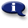# .

## Syllabus Information

Fall 2022
May 31,2023Use this page to maintain syllabus information, learning objectives, required materials, and technical requirements for the course.

Syllabus Information
MTH 098 - Math Literacy
Associated Term: Fall 2022
Learning Objectives: Upon successful completion of this course, the student will be able to:
1. Interpret quantitative information presented numerically, verbally or graphically
2. Create and use mathematical models
3. Demonstrate critical thinking in quantitative settings
4. Express mathematical ideas vocally and in writing
5. Apply mathematical skills with civic, environmental, and ethical awareness
6. Make appropriate use of calculators and spreadsheet software
7. Use ratios, rates, and percents to compare quantities
8. Use proportional reasoning to solve problems
9. Evaluate expressions and use formulas in a variety of contexts
10. Express numbers with scientific notation
11. Convert quantities using dimensional analysis
12. Use rates of change and percent increase and decrease to describe changing quantities
13. Write linear and exponential models. Recognize them in graphical and numerical contexts
14. Select a graph for presenting a given data set
15. Calculate a mean or weighted mean in a given context
Required Materials:
Technical Requirements: